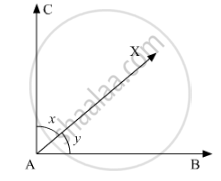# Define Complementary Angles. - Mathematics

Define complementary angles.

#### Solution

Complementary Angles: Two angles, the sum of whose measures is 90°, are called complementary angles.

Thus, angles ∠BAX and ∠XACare complementary angles, if

x +y = 90°Example 1:

Angles of measure 50°and 40°are complementary angles, because

50°+40° =90°

Example 2:

Angles of measure 60°and 30°are complementary angles, because

60°+30° =90°

Concept: Introduction to Lines and Angles
Is there an error in this question or solution?

#### APPEARS IN

RD Sharma Mathematics for Class 9
Chapter 10 Lines and Angles
Q 1 | Page 50